# Supporting the TEKS Mathematical Processes with Exemplars Performance Tasks and Rubric at the Third Grade Level

Written By Exemplars Math Consultants: Deborah Armitage, M.Ed. and Dinah Chancellor, M.Ed.

###### Summer Blog Series Overview:

Exemplars performance-based material is a supplemental resource that provides teachers with an effective way to implement the Math TEKS through problem solving. This blog represents Part 4 of a five-part series that features a problem-solving task linked to a Math TEKS Unit of Study and a student’s solution in grades K-5. Evidence of all seven Mathematical Process Standards will be exhibited by the end of the series.

The Exemplars Standards-Based Math Rubric allows teachers to examine student work against a set of analytic criteria that consists of the following categories: Problem Solving, Reasoning and Proof, Communication, Connections and Representation. There are four performance/achievement levels: Novice, Apprentice, Practitioner (meets the standard) and Expert. The Novice and Apprentice levels support a student’s progress toward being able to apply the criteria of a Practitioner and Expert. It is at these higher levels of achievement where support for the Mathematical Processes is found.

Exemplars problem-solving tasks provide students with an opportunity to apply their conceptual understanding of standards, mathematical processes and skills. Observing student anchor papers with assessment rationales that demonstrate the alignment between the Exemplars assessment rubric and the Math TEKS can be insightful for educators. Anchor papers and assessment rationales provide examples of what to look for in your own students’ work. Examples of Exemplars rubric criteria and the Mathematical Processes are embedded in the assessment rationales at the bottom of the page. The full version of our rubric may be accessed here. It is often helpful to have this in hand while reviewing a piece of student work.

#### Blog 4: Observations at the Third Grade Level

The fourth anchor paper and set of assessment rationales we’ll review in this series is taken from a third grade student’s solution for the task, “Henry’s Lego Structure.” This task is one of a number of Exemplars tasks aligned to the Algebraic Reasoning Unit.

“Henry’s Lego Structure” could be used toward the end of the learning time allocated to this Unit. This particular task provides third graders with an opportunity to apply different strategies to determine how many Legos are needed to build a three-level structure and if “Henry” has enough Legos to build a fourth level. Students need to bring an understanding of the terms twicethree times and pattern to the task as well as the correct calculation. When assessing this task, teachers can observe which forms of calculation a student chooses to use and if s/he can solve a two-step problem.

There are a variety of strategies for students to consider in forming their solutions. Some examples include using actual Legos to model the structure, diagramming the structure, creating a table, tally chart or using a number line.

Henry wants to build a structure with his new Lego set. The Lego set contains five hundred Legos. The structure will be three levels high. The first level is made of twenty-seven Legos. Henry uses twice as many Legos for the second level as for the first level. Henry uses three times as many Legos for the third level as for the second level. How many Legos does Henry use to build his structure with three levels? If this pattern continues, does Henry have enough Legos in his new set to build a fourth level on his structure? Show all of your mathematical thinking.

### Math TEKS Alignments:

###### Exemplars Algebraic Reasoning Unit

The Algebraic Reasoning Unit involves analyzing numerical patterns and the relationships between addition and subtraction; multiplication and division in order to answer questions such as:

• What models can be used to represent addition and subtraction situations?
• What models can be used to represent multiplication and division situations?
• Given a multiplication or division situation, how can you generate an equation in which the unknown is either a missing factor or a missing product?
• Given an equation with a missing factor or a missing product, how can you create a situation to match it?
• How can a table of real-world number pairs help to generate multiplication or division equations to represent the relationships in the table?

The standards covered in this Unit include:

3.5 Algebraic Reasoning:

• 3.5A represent one- and two-step problems involving addition and subtraction of whole numbers to 1,000 using pictorial models, such as strip diagrams and number lines, and equations.
• 3.5B represent and solve one- and two-step multiplication and division problems within 100 using arrays, strip diagrams, and equations.
• 3.5C describe a multiplication expression as a comparison such as 3 x 24 represents 3 times as much as 24.
• 3.5D determine the unknown whole number in a multiplication or division equation relating three whole numbers when the unknown is either a missing factor or product such as the value 4 makes 3 x [ ] = 12 a true equation.
• 3.5E represent real-world relationships using number pairs in a table and verbal descriptions such as 1 insect has 6 legs, 2 insects have 12 legs, and so forth.
###### Mathematical Process Standards
• 3.1A apply mathematics to problems arising in everyday life, society, and the workplace;
• 3.1B use a problem-solving model that incorporates analyzing given information, formulating a plan or strategy, determining a solution, justifying the solution, and evaluating the problem-solving process and the reasonableness of the solution;
• 3.1C select tools, including real objects, manipulatives, paper and pencil, and technology as appropriate, and techniques, including mental math, estimation, and number sense as appropriate, to solve problems;
• 3.1D communicate mathematical ideas, reasoning, and their implications using multiple representations, including symbols, diagrams, graphs, and language as appropriate;
• 3.1E create and use representations to organize, record, and communicate mathematical ideas;
• 3.1F analyze mathematical relationships to connect and communicate mathematical ideas; and
• 3.1G display, explain, and justify mathematical ideas and arguments using precise mathematical language in written or oral communication.
1 / 6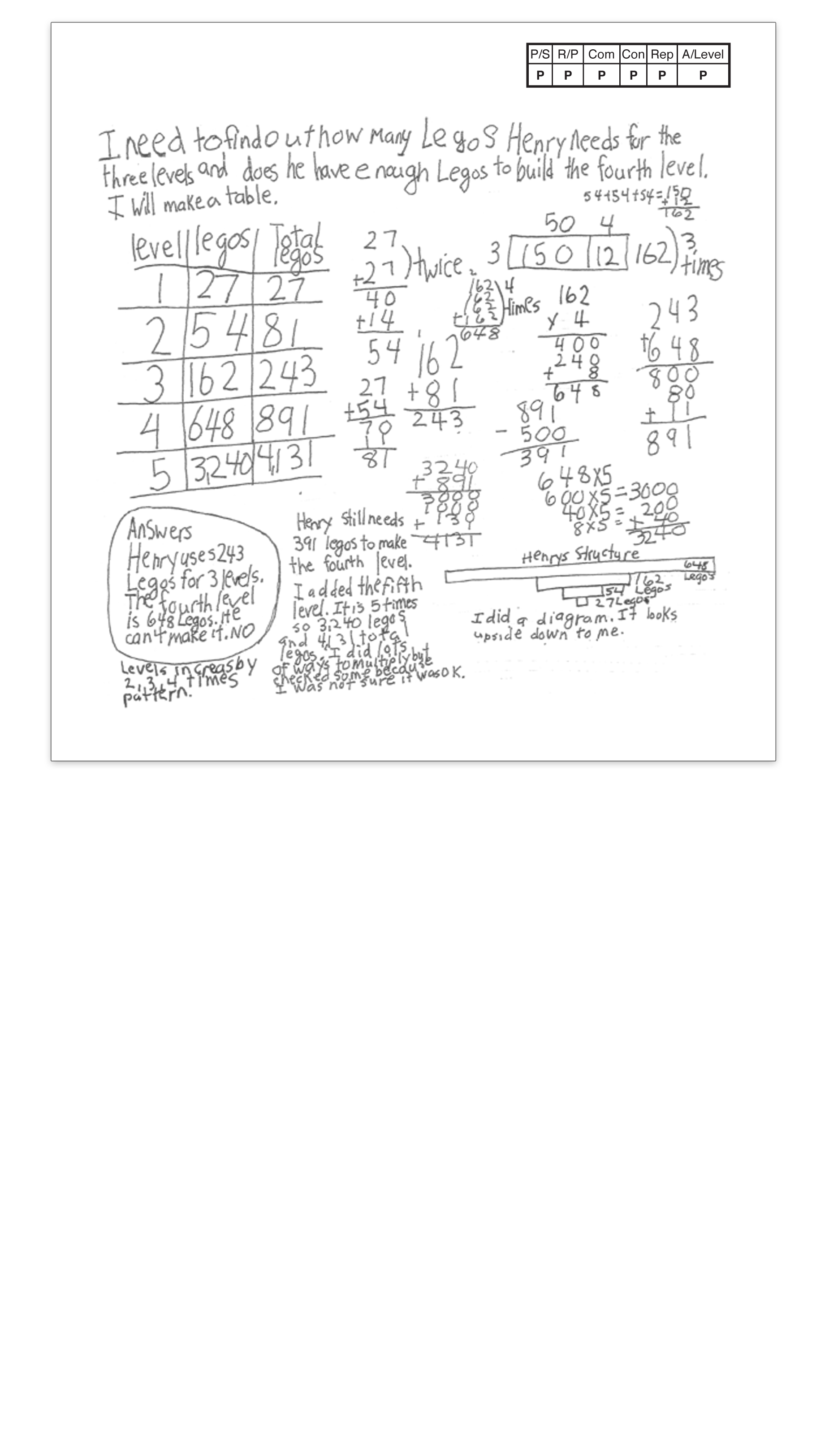2 / 6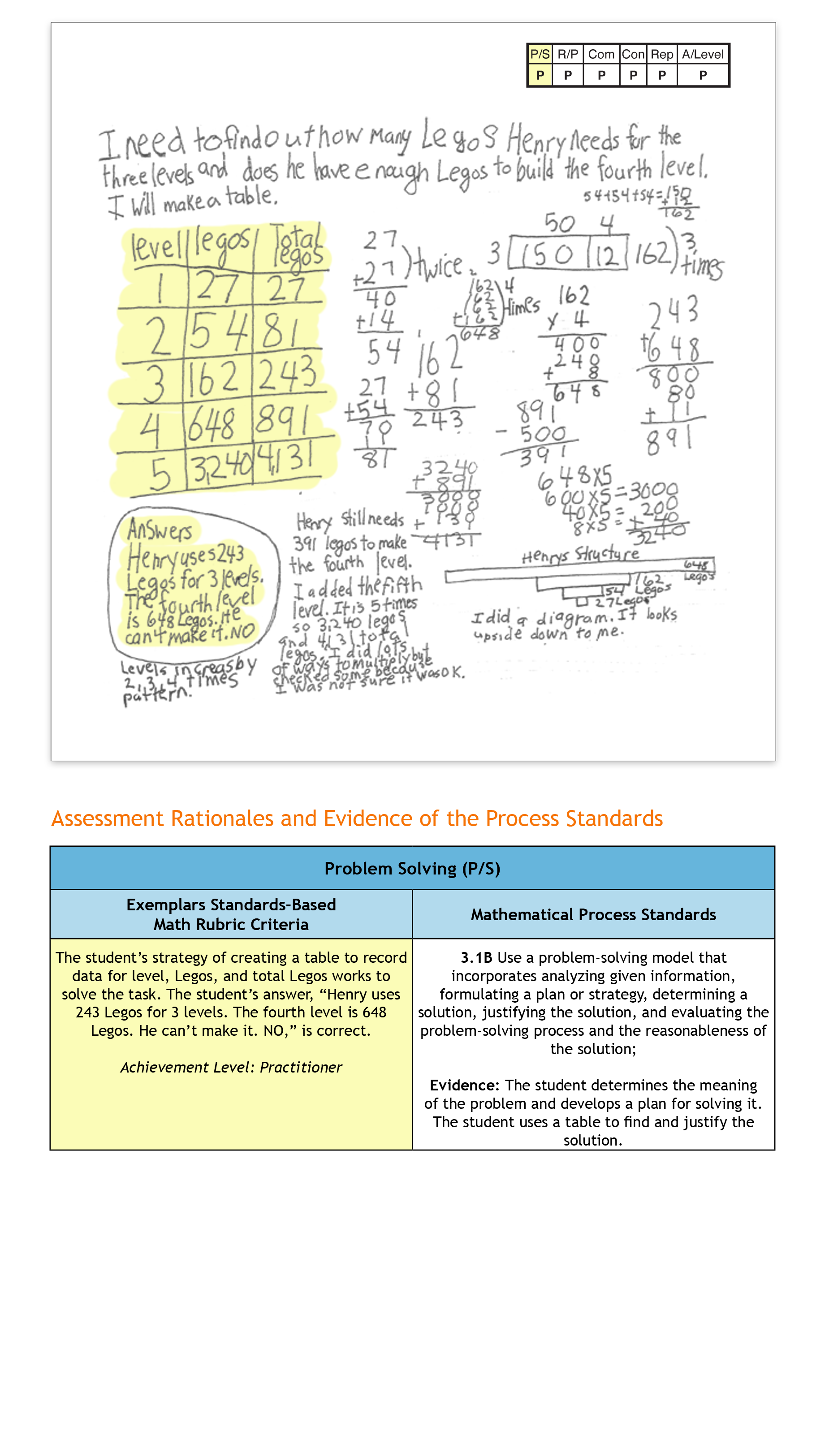3 / 6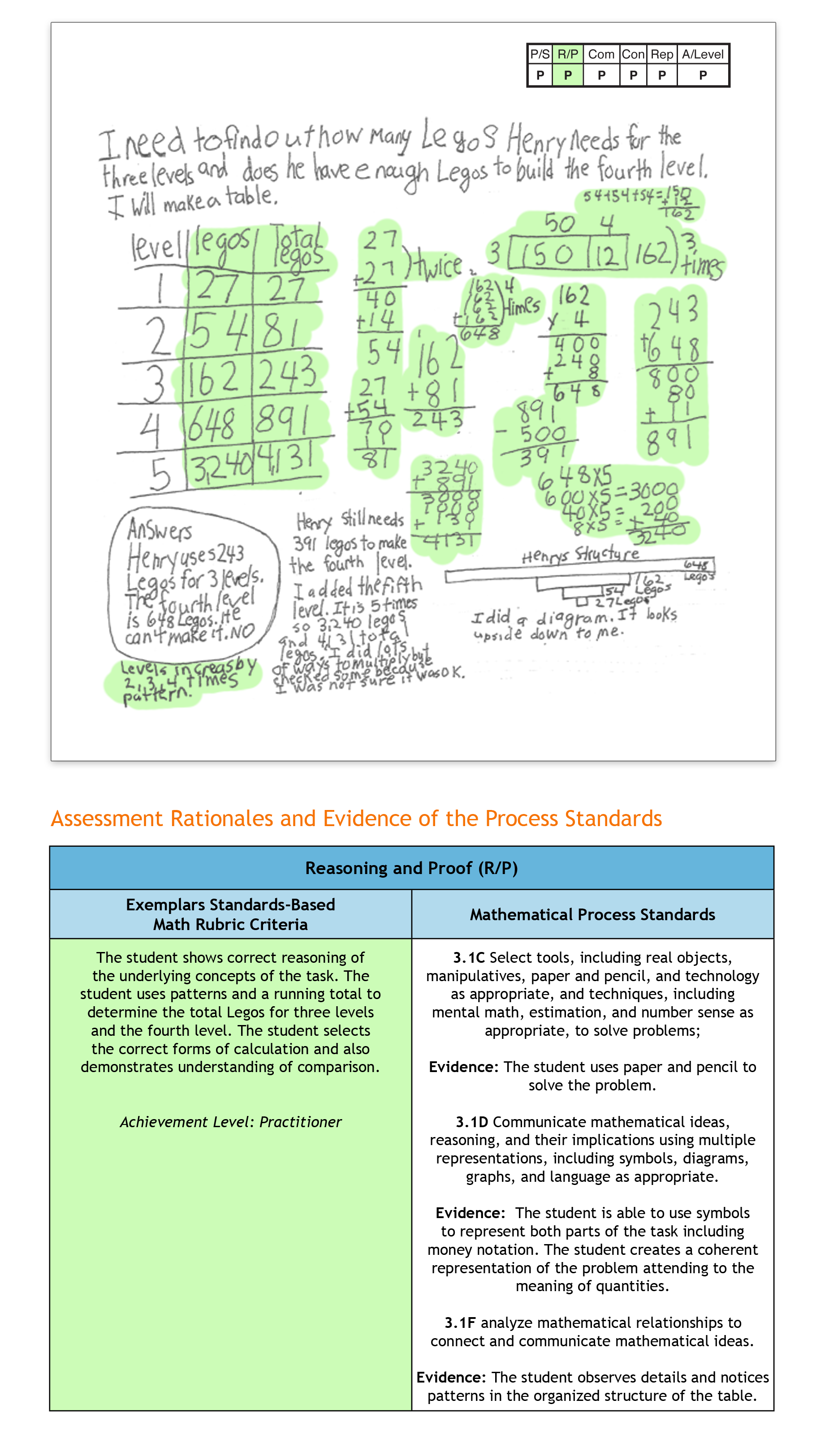4 / 6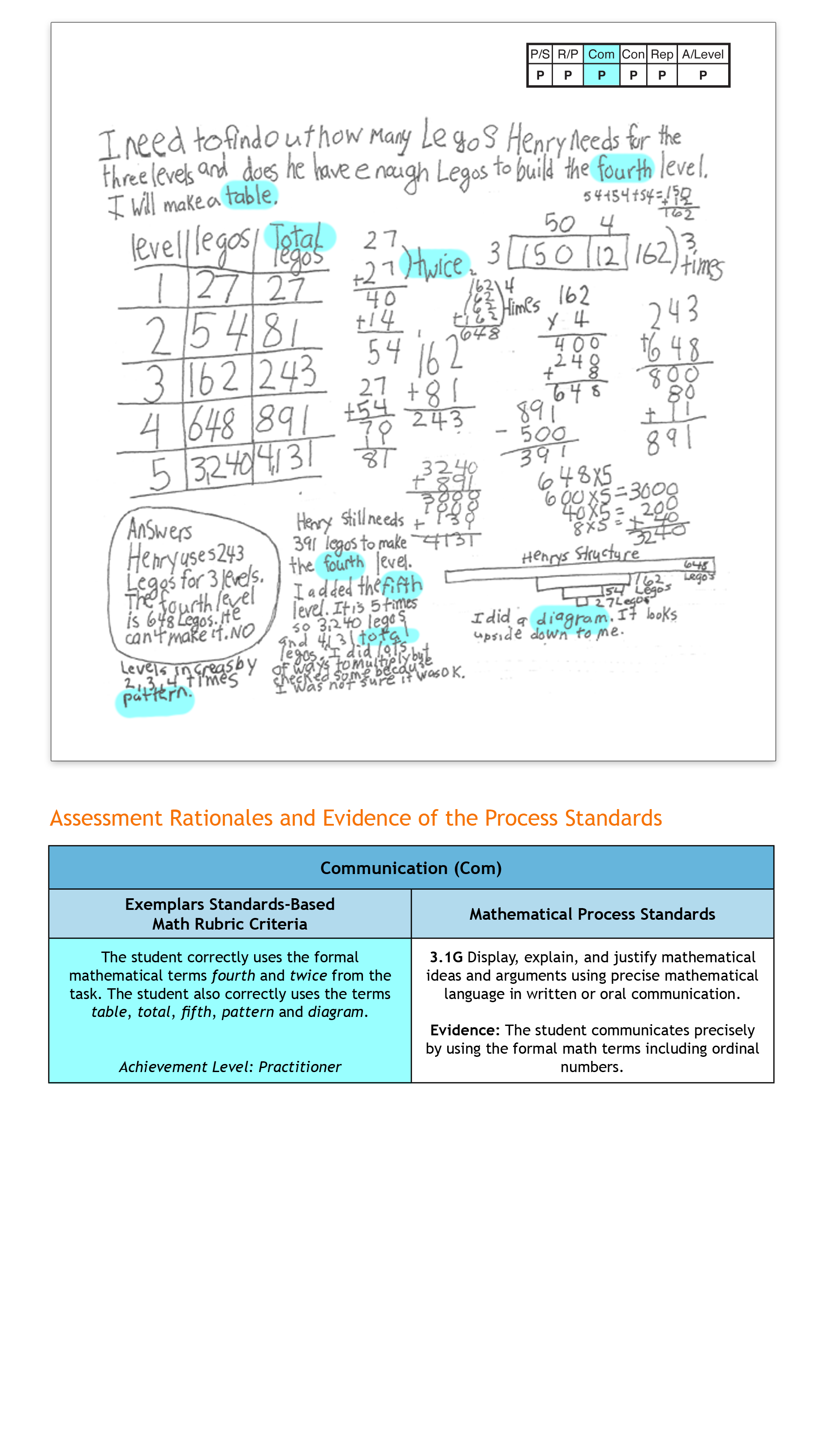5 / 6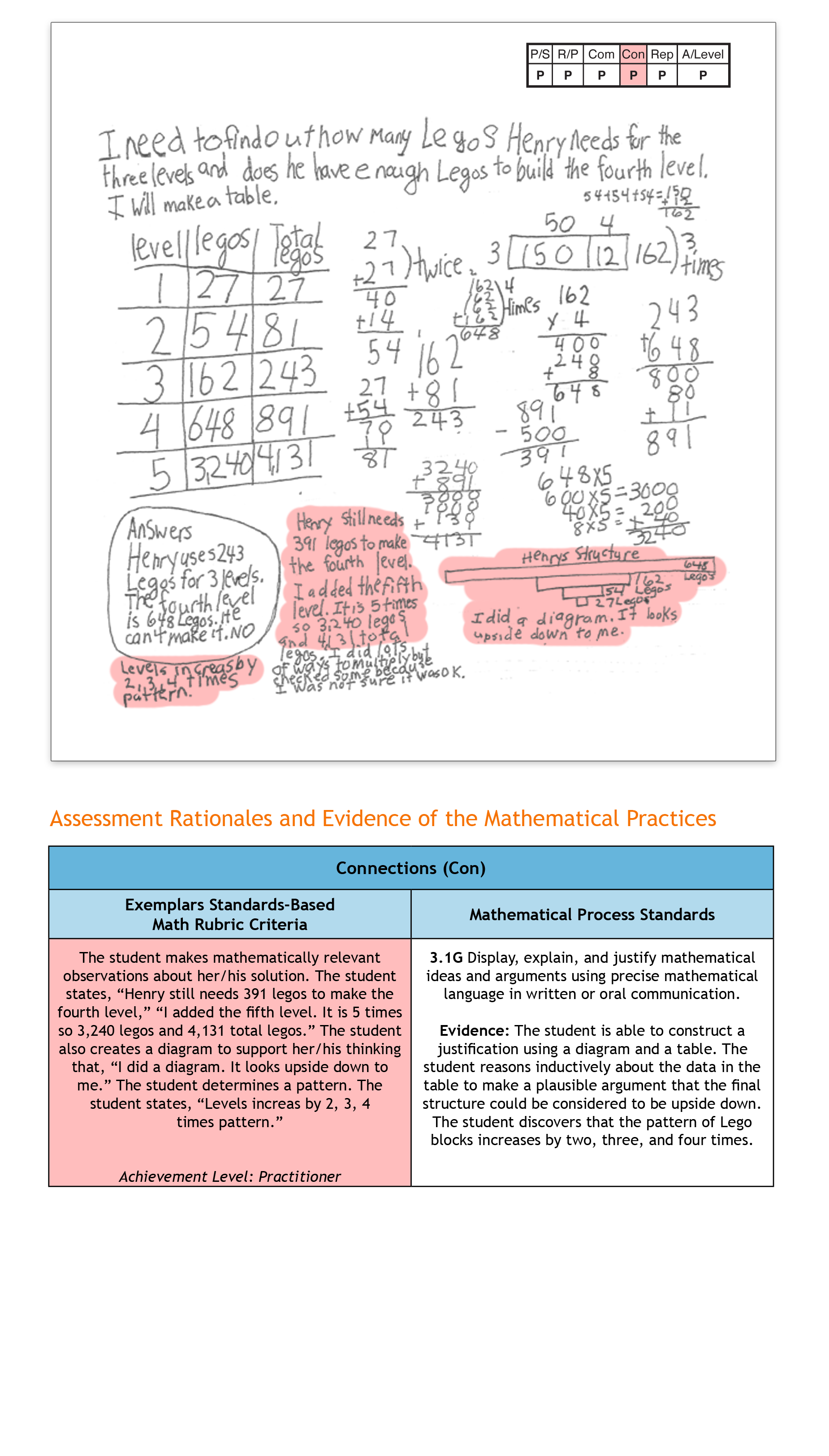6 / 6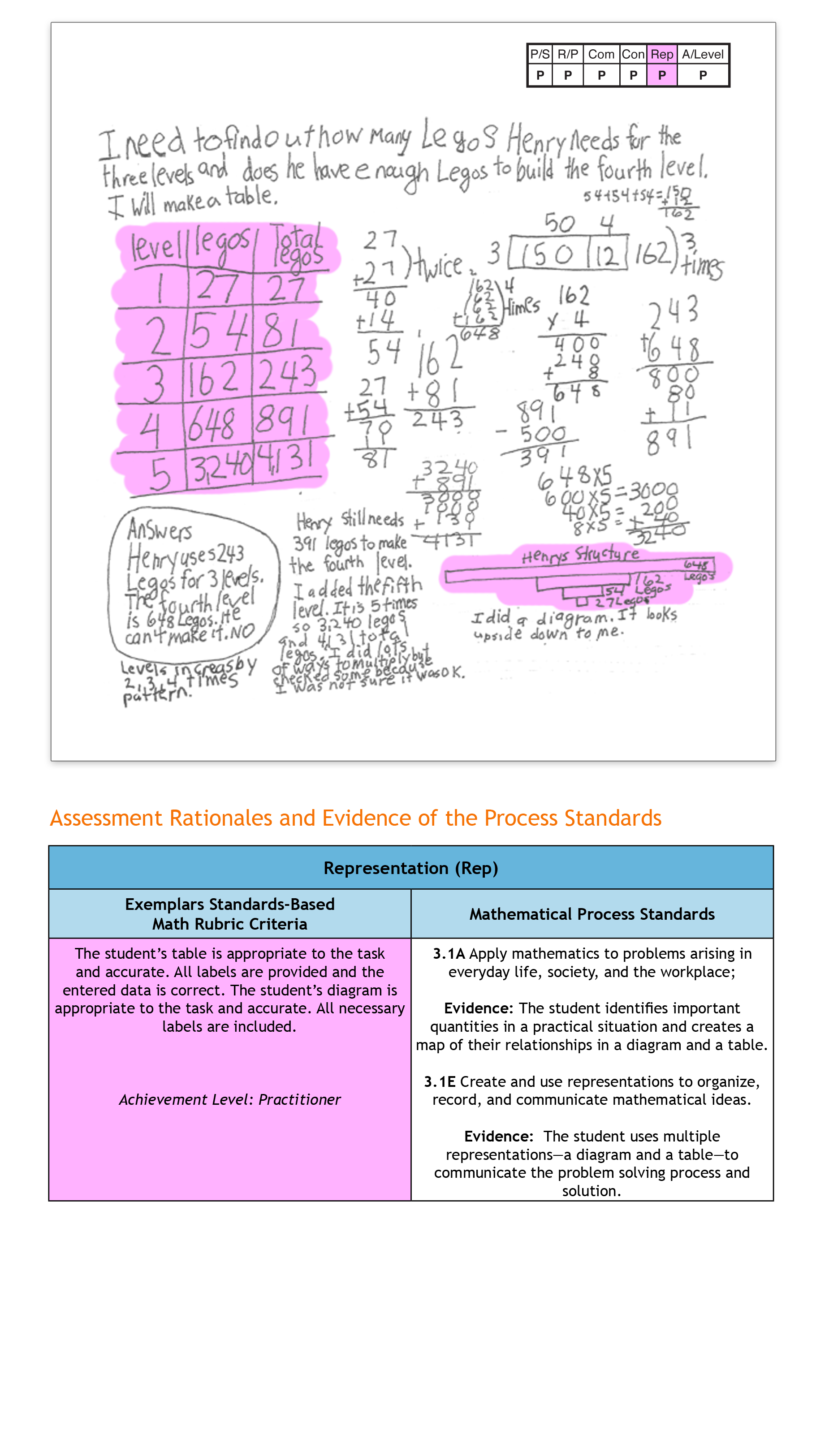Topics: